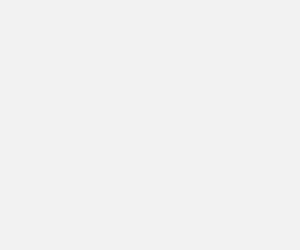×The Free 30-Day CAT RC Course

"It is designed to help you excel in the upcoming CAT 2023 exam"

-By Lavleen Kaur Kapoor. Over 1,73,000 Subscribers

No thanks >

OPEN

OPEN

## IBSAT, CAT, XAT, NMAT, GMAT

OPEN#### Get Personalized MBA Mentorship

Connect with my team on WhatsApp for unbiased MBA College Selection advice.

# Expert Q&A on DI

Exam Registration: 17th March 2022 to 20th April 2022MBA aspirants, who will be appearing in MAH CET exam on April, 2022 may Study following Q& A on DI:

The following pie-chart shows the percentage distribution of the expenditure incurred in publishing a book. Study the pie-chart and the answer the questions based on it.

Various Expenditures (in percentage) Incurred in Publishing a Book1)
If for a certain quantity of books, the publisher has to pay Rs. 30,600 as printing cost, then what will be amount of royalty to be paid for these books?

A.   Rs. 19,450         B.   Rs. 21,200
C.   Rs. 22,950         D.   Rs. 26,150

Explanation:

Let the amount of Royalty to be paid for these books be Rs. r
Then, 20:15 = 30600:r
r = Rs. 30600 x 15    = Rs. 22,950.
20
2)
What is the central angle of the sector corresponding to the expenditure incurred on Royalty?

A.   15º          B.   24º
C.   54º          D.   48º

Explanation:

Central angle corresponding to Royalty          = 15% of 360º

=  15    x 360º
100

= 54º.
3)
The price of the book is marked 20% above the C.P. If the marked price of the book is Rs. 180, then what is the cost of the paper used in a single copy of the book?

A.   Rs. 36         B.  Rs. 37.50
C.   Rs. 42         D.  Rs. 44.25

Explanation:

Clearly, marked price of the book = 120% of C.P.
Also, cost of paper = 25% of C.P
Let the cost of paper for a single book be Rs. n.
Then,                120: 25 = 180: n
n = Rs.  25 x 180 = Rs. 37.50
120

4)
If 5500 copies are published and the transportation cost on them amounts to Rs. 82500, then what should be the selling price of the book so that the publisher can earn a profit of 25%?

A.  Rs. 187.50            B.  Rs. 191.50
C.  Rs. 175.00            D.  Rs. 180.00

Explanation:

For the publisher to earn a profit of 25%, S.P. = 125% of C.P.
Also Transportation Cost = 10% of C.P.
Let the S.P. of 5500 books be Rs. x.
Then,         10 : 125 = 82500 : x
x = Rs.   125 x 82500   = Rs. 1031250.
10

S.P. of one book = Rs. 1031250   = Rs. 187.50
5500

5)
Royalty on the book is less than the printing cost by:

A.  5.0%            B. 33.5%
C.  20.0%          D.  25.0%

Explanation:

Printing Cost of book = 20% of C.P.
Royalty on book = 15% of C.P.
Difference = (20% of C.P.) - (15% of C.P) = 5% of C.P.
Percentage difference     =     Difference    x 100%
Printing Cost

=      5% of C.P.     x 100%
Printing Cost

=     25%.

Stay informed, Stay ahead and stay inspired with MBA Rendezvous